Spare Time Labs 2.0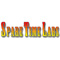WelcomeEazyCNC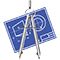jDraft 2.0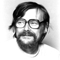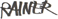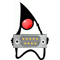PureJavaComm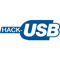PIC CDC ACM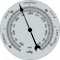Weather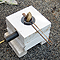Ten-Buck FurnaceH8S Bootloader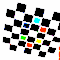Camera Calibration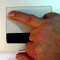Multitouch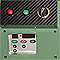Myford VFD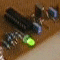Fun with HC08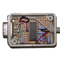bl08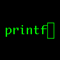printf II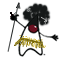Java Goes Native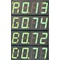Densitometer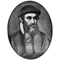printf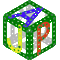jApp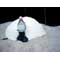Igloo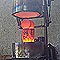New Furnace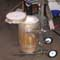New Furnace
Part II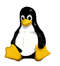Linux 101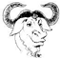H8S/gcc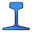Quickie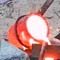Gas Fired Furnace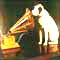Down Memory Lane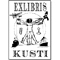Exlibris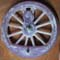Wheel Patterns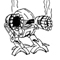Glitches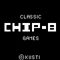CHIP-8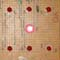eDiceAnimato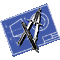jDraftJNA Benchmark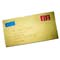Contact Info
Created 7.4.2006 (TEST)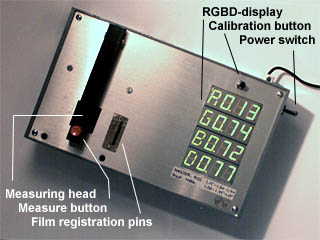# Densitometer

## Logarithms on a Budget

(image courtesy of Jan-Erik Nystrom)

This Friday I happened to be stranded for a few hours with my computer in a hospital cafeteria with nothing better to do so I started cleaning up my hard-drive. And look what I found!

The Source Code of an old project, a Densitometer , that Jankke and I did back in 1999, written in 68HC11 Assembly Language .

In those days film (as opposed to today's digital photography) was still very much used and Jankke often needed a densitometer in his work. Being a tinker for all things mechanical and electronic he wanted to build one so I volunteered to write the software.

In case you do not know, densitometer is a device to measure the optical density , that is darkness, or properly speaking transparency (those with a University Degree would call it transmittance I guess) of a film. As the human eye has a logarithmic response to light, this [density] is most conveniently defined as the logarithm of the inverse of transparency.

Why is the eye responce logarithmic ?

Well, so many things in nature are logarithmic, but my personal thinking is that because the Designer or Evolution way back in the Cambrian Explosion times made the eye that way. Possibly because the diminishing of light as it travels through materia is a sort of exponential process. So a logarithmic response makes relatively equal changes appear as linearly equal changes which helps us in our everyday life to zoom on the pray or avoid the predator, I guess.

But alas, I digress, back to densitometry.

To measure the density of a film you use a light source and you measure the light intensity with and without the film between your meter and the light source. The density is the logarithm, base ten by definition, of the ratio of those measurements. Simple.

Now this finally brings me to the point of this page, i.e how to do the logarithmic conversion with the limited computation resources of Jankke's Densitometer. He used (to give credit where it is due, Jankke did the concepting, schematics and construction, Chrisse found the sensor chip, I just did the software) a Motorola 68HC11 because we were familiar with it and we had a few extra parts laying around. This particular model had 512 bytes of RAM and 2048 bytes of EEPROM. And that's it, no Flash, no nothing. Those were the days.

One Friday evening I started to work on this project.

Jankke's orignal idea was to do the logarithmic conversion with integer multiplications only. Remember, the logarithm is the answer to the question of "to which power the base number needs to be raised to the get to the number in question". And "raising to a power" is of course simply multiplying a number by itself. The required density resolution is 0.01 OD (Optical Density), so the question becomes how many times 10**0.01 needs to be multiplied by itself to equal the transmittance ratio. Work that out by trial and the answer is the density in units of 0.01 OD i.e. multiplied by 100.

For example:

100.01 ~ 1.023292992

So, knowing that a film of density 0.3 passes through about half of the light we can verify:

1.023239 (0.3 * 100) ~ 1.995

I spent a few minutes toying with the idea but did not fancy it. Figuring out the required numeric range and how to implement that 'simple' solution with integer math left me with a feeling that this simple cure for this math problem was worse than the actual disease. So I considered what else I could do.

If only we had a litle bit (ha ha) more memory ... hmm...the C-compiler for HC11 that I was using at work had floating point math with trig functions and it only required (IIRC) about 4 kB. But adding that memory would require running the chip in expanded mode and wiring the external bus, not very attractive, especially as the hardware was already there. Curse Jankke for being so fast and my big mouth for promising to fit this software into the internal EEPROM.

Respecting the compiler math package writers enough to understand that they had done their homework I realised that it would probably be futile to try and squeeze all that math into 2 kB. Even I am not that good a hacker ;-)

Then it occurred to me that in working with floating point math the actual accuracy required is only about four digits, so how about a tiny floating point package with only that much precision? Say 8 bits for the exponent and sign and 16 bits for the mantissa (which means "minor addition", what a joke!). This would make the basic integer arithmetic operations in the math package simple, as only 16 bit arithmetics would be required and the HC11 mcu has 8x8 bit multiply and 16/16 division instructions.

I had once contemplated writing a floating point package for my Telmac, so I knew that it is not such a big task after all and the C-compiler came with trig math package source code....here it is, note that it may not be, although I think it is, 100% ok because the code was salvaged from the assembly code comments.

``````
#define v05 0.500000
#define v07 0.707092
#define a0 -64.123047
#define a1 16.383789
#define a2 -0.789551
#define b0 -769.484375
#define b1 312.031250
#define b2 -35.667969
#define c1 0.693359
#define c3 0.693146

float log(float ARG)
{
float FRACT, NUM, DEN, SQ, ZUM, FA, FB, QR;
int INT;
FRACT = fract(ARG,&INT);
NUM = FRACT - v05;
if (FRACT > v07) {
NUM = NUM - v05;
DEN = FRACT * v05 + v05;
}
else {
DEN = NUM * v05 + v05;
--INT;
}
ZUM = NUM / DEN;
SQ = ZUM * ZUM;
FA = a0 + SQ * (a1 + SQ * a2);
FB = b0 + SQ * (b1 + SQ * (b2 + SQ));
QR = ZUM + ZUM * SQ * FA / FB;
FA = INT;
return FA * c3 + QR;
}
``````

But that would still be quite a big task to debug without proper tools.

Then I got a brain wave: I could write a C++ class to mimic the built in float type using my own low precision floating point. This would enable me to use the original C-code for the logarithm and enable me to debug the floating point math on my Mac.

In just a few hours I had a more or less working C++ class that did floating point math and logarithms using 16 bits for the mantissa (four decimal digits accuracy) and 8 bits for the exponent and sign. Pity I do not seem to be able to find that piece of code anymore, it's bound to be somewhere but can't lay my hands on it right now.

Translating that to HC11 assembly language was pretty trivial a task.

To debug the assembly code I transferred the code onto an old x-ray film printer keyboard (called Autoprint) that had a 2 x 40 character LCD display and happened to use the same 68HC11 chip. The display enabled me to compare intermidiate results from the assembly code and the C++ floating point package and in a few hours the whole thing was up and running.

Adding the user interface and the measurement code was piece of cake and by Sunday evening it was all done!

Nowadays, when the smallest of processors contains kilobytes of Flash memory and you can program them in C this source code has little use or value but I'm publishing it here in case someone finds it interesting or educational.

Although the code is obsolete, the strategy used in implementing it is still valid.

In that spirit some people may find the following description of the floating point a useful companion to the obsolete source code ;-)

Floating point math is pretty easy although it took a long-ish time to develop and perfect it to the IEEE standard we all know and love. Thank you Kahan and Intel!

An interesting trivia is that von Neuman himself refused to include floating point math in 1946 because he thought that the 'modest' advantage of floating point numbers would be outweighted by the cost of the additional bits needed to store the numbers.

In floating point math a real number is represented with two integers, mantissa and exponent.

Note that in the following I've used the 'programmers' notation where multiplication and division have equal precedence and evaluate from left to right. Also note that the C-code samples are just sketches not actual debugged code.

The mantissa contains the significant numbers and the exponent is a scale factor which is expressed as the
power of two by which to multiply the mantissa to get the actual value:

value = mantissa / divisor * 2exponent

In C you could express a this 'real' number as follows:

``````
typedef struct{
int sgn : 1;
int exp : 7;
int mantissa : 16;
} real;
``````

The divisor is an implied constant which allows us to express mantissa as a simple integer value. Because it is a constant it is not necessary to store it with the number. By selecting a power of two the division can be implemented as shifts and by selecting it to be 216 the shift is equal to just picking the hi word of a 32 bit value.

To maximize accuracy we always try to keep the mantissa so that it utilizes as many bits of that integer mantissa as possible, in other words as large as will fit in the 16 bit integer mantissa. This representation (when mantissa is between 0.5 and 1.0 ) is called the normalized form.

In C normalization would go something like this:

``````
real normalize(real res) {
while ((res.mantissa & 0x8000)==0) {
res.mantissa <<= 1;
exp--;
}
return res;
}
``````

Let's do some numbers, it's easier to understand that way. Say we want to express the value 3.14159 as a floating point number in this 8 bit exponent 16 bit mantissa format I envisioned. In this format mantissa the implied divisior is 216 = 65536 which represents a value of 1.0 (note that you can't actually do that because it would overflow the 16 bits of the mantissa). So the mantissa for 3.14159 becomes (integer math, remember):

3.14159 * 216 / 216 = 205887 / 65536

and the scale factor is (by default):

1 = 20 giving exponent of 0

But wait, the mantissa is larger than 16 bits! So we need to fix this by giving up some accuracy by changing the scaling. This is called normalizing.

This is simple, every time the mantissa divides by two you compensate by multiplying the scale factor by two, which simply means incrementing the exponent by one. So we get:

205887 / 216 * 20 = 102943 / 216 * 21 = 51471 / 65536 * 22

which works out to:

0,785385132 * 4 = 3,141540527

Pretty close, huh?

Turn those numbers (51471 and 2) into hex (C90F, 02) and reverse the order and you know that the value PI in my 16+8 floating point format is in binary hex 42C90F.

You may wonder where that extra '4' came in that number, should it not have been 02C90F?

That is because I use the same clever trick that most floating point formats use. In my floating point format the mantissa takes full 16 bits so the remaining 1 byte needs to store the sign and exponent. This leaves 7 bits for the exponent. 27 is 128 so the exponent range is -64 .. 63. Which could be stored 'as is' in 2's complement format, but the exponent is stored 'biased' by adding 64 (hence hex 40 and the extra '4'). Stick the sign bit of the mantissa in the front and you get a 24 bit number of the form [sign][exponent + 64][mantissasa].

And this is where it gets exciting: it turns out that comparison (which larger or equal) can be done by simply doing an integer 2's complement comparison! Also zero is zero which is a bonus in terms of efficiency.

Simple, efficient and neat - I wish I had thought all this out by myself!

What is nice about this form is that it allows you to express both large and small numbers with less bits than pure
integer math. And faster too, strange as it may seem.

A proper IEEE format uses some clever design to squeeze every little bit of accuracy and mathematical niceties out of the
bits of mantissa and exponent but that was not necessary for me.

Ok, now we know the format, how about math.

That's pretty simple, take multiplication for example, given two floating point numbers A and B both with mantissa (call them MA, MB) and exponent (call it EA,EB) we get:

result = MA / 216 * 2EA * MB / 216 * 2EB

Rearrange:

result = MA * MB / (216 * 216) * 2EB * 2EA

Simplify:

result = MA * MB / 216+16 * 2(EB + EA)

We realise that mantissa is just 16 x 16 bit integer multiplication and the exponents just need to be added together. The multiplication result is of course 32 bits but we need only the 16 most significant bits which we get by picking/computing just the hi word, not even a shift involved.

In C this would go along the lines of:

``````
real multiplication(real arg1, real arg2) {
real res;
res.mantissa = (arg1.mantissa * arg2.mantissa)>>16;
res.exp = arg1.exp + arg2.exp - 64;
res.sgn = arg1.sgn ^ arg2.sgn;
return normalize(res);
}
``````

Now if that is not cool I do not know what is!

All we need to do is to normalize and truncate the mantissa as 16 x 16 bits give you 32 bits of result. Actually, if we do not allow denormalized numbers then we do not even need to perform full 16 x 16 bits multiplication, the lowest bits of the 32 bit result cannot contribute to the result.

What about addition? Surprisingly this is a little bit more complicated.

Of course the starting point is the same:

result = MA / 216 * 2EA + MB / 216 * 2EB

But now we can't re-arrange to gain anything, because we need to do the scaling first. This could be easily done with shifts until, say, both exponents are zero. But that would be wasteful, instead it is easy to realize that there is a common factor which is the smaller of the exponents. Suppose it is the exponential part of B in this example and we get:

result = (MA / 216 * 2EA / 2EB + MB / 216) * 2EB

which simplifies to:

result = (MA * 2(EA - EB) + MB) / 216 * 2EB

So we see that it is only necessary to shift left the mantissa of the larger of the values to the left EA - EB times. (Actually it makes sense to shift the other value to the right and lose those bits that fall off the right edge, because they will not be contributing to the result after normalization.)

After 'aligning' the mantissas we just do an integer addition.

The common factor (exponent of B) is the same as the exponent of the result. The only thing that is left is to leave out the 'extra bits' of the addition. Before we can do that we need to normalize the result as explained above.

So floating point addition involves just some integer additions, substraction and shifts. Not quite as neat as multiplication, as evidenced by this C sketch:

``````
real addition(real arg1, real arg2) {
real res;
if (arg1.exponent < arg2.exponent) {
real t= arg1;
arg2 = arg1;
arg1 =t;
}
res.exp=arg1.exp;
arg2.mantissa = (arg2.mantissa) >> abs(arg2.exp-arg1.exp)
if (arg.sign == arg2.sign)
res.mantissa= arg1.mantissa + arg2.mantissa;
else {
if (arg1.mantissa > arg2.mantissa) {
res.mantissa = abs(arg1.mantissa - arg2.mantissa);
res.sign=arg1.sign;
}
else {
res.mantissa = abs(arg2.mantissa - arg1.mantissa);
res.sign=arg2.sign;
}
}
return normalize(res);
}
``````

That is about all, I'm sure there are better tutorials on the web but I'm unable to check at the moment 'cause this hospital cafeteria has no WLAN access.

Hope this helps you to follow the source.

cheers Kusti

19.6.2006